# RD Sharma Solutions For Class 7 Maths Chapter 15 Properties of Triangles Exercise 15.4

The PDF of RD Sharma Solutions for Class 7 Exercise 15.4 of Chapter 15 Properties of Triangles is given below. Learners can access and download the PDF for free. The solutions to this exercise come with detailed explanations structured by our expert teachers that further makes learning and understanding of concepts an easy task. RD Sharma Solutions for Class 7 textbook includes the concepts in a detailed and step by step way to help the students understand effectively. This exercise includes the triangular inequality property. Based on this theorem, questions are prepared.

## Download the PDF of RD Sharma Solutions For Class 7 Maths Chapter 15 – Properties of Triangles Exercise 15.4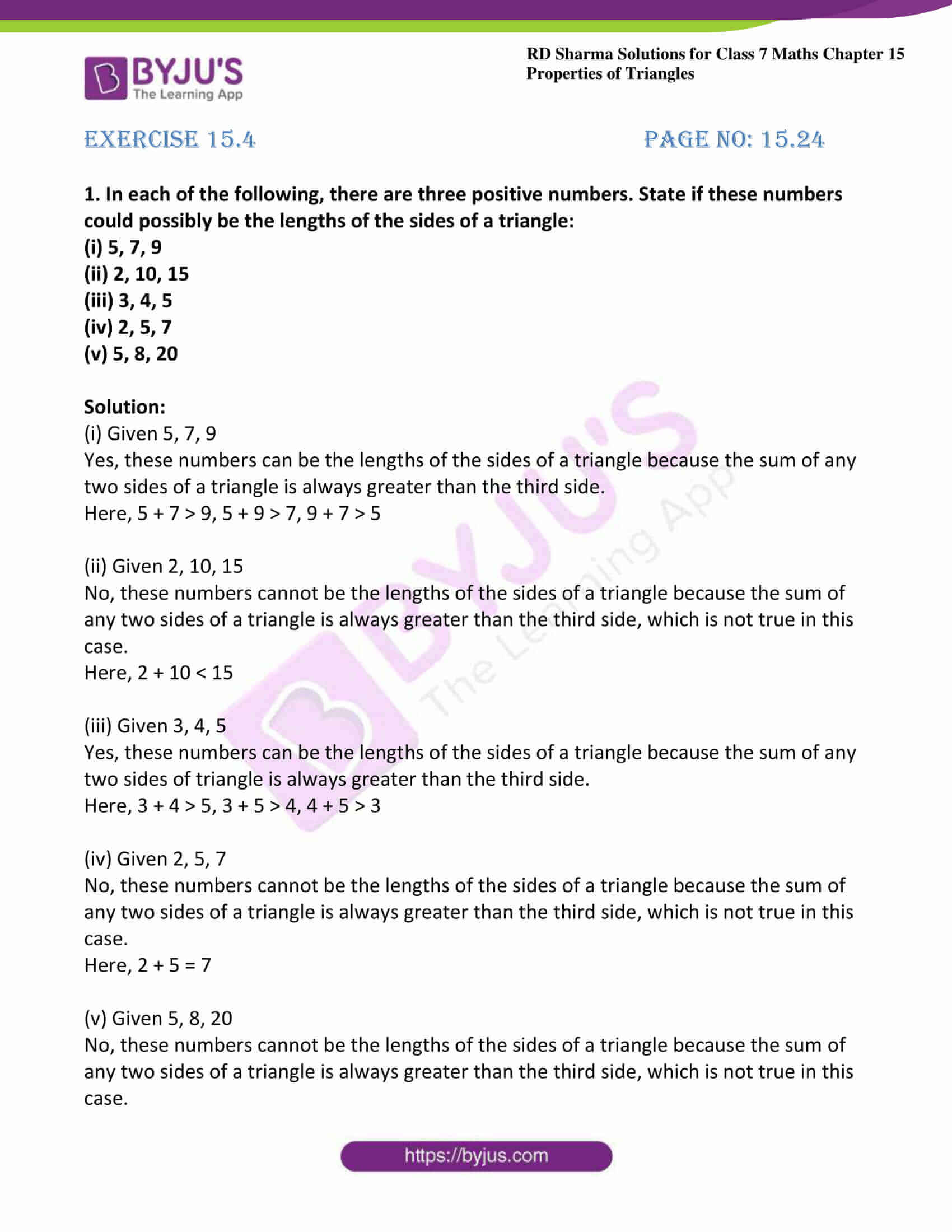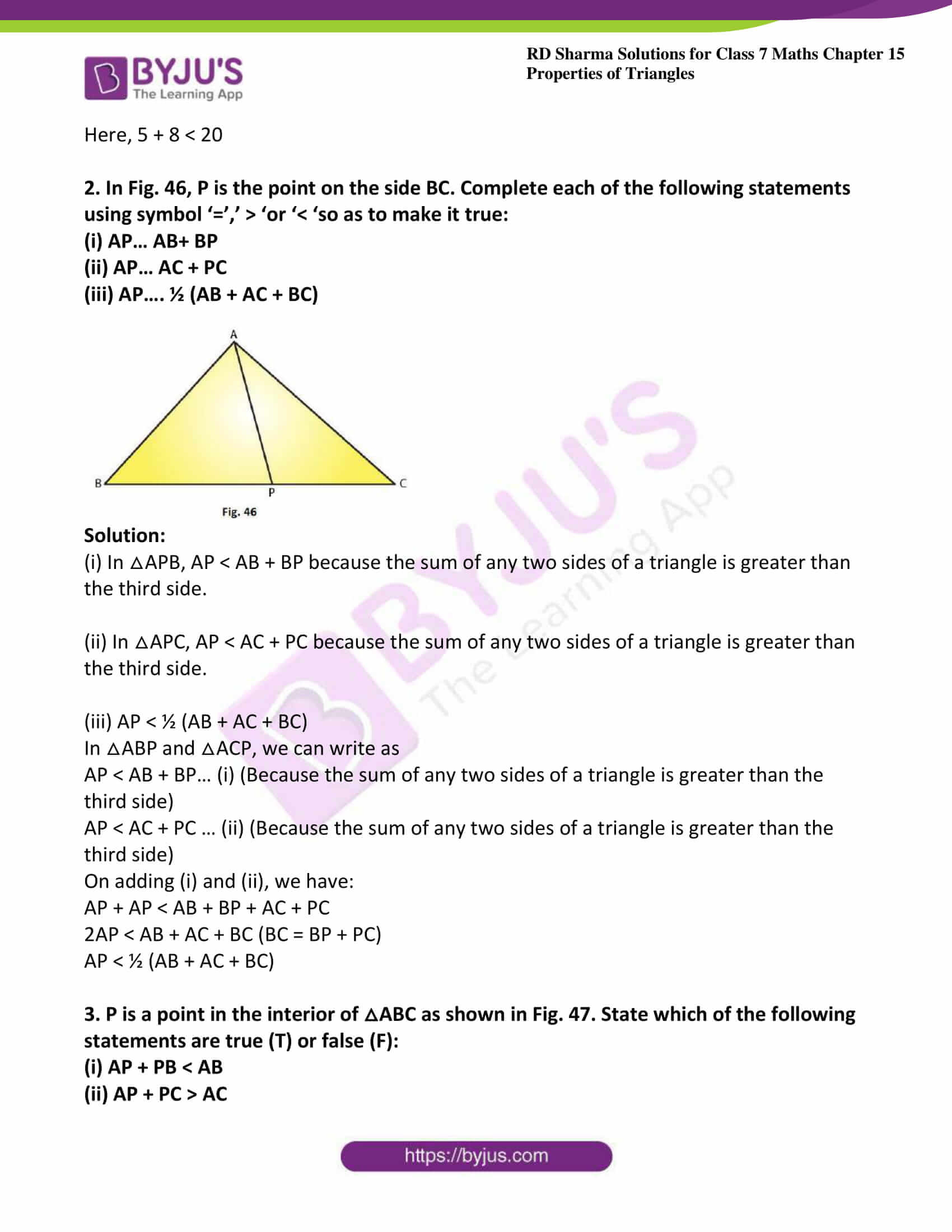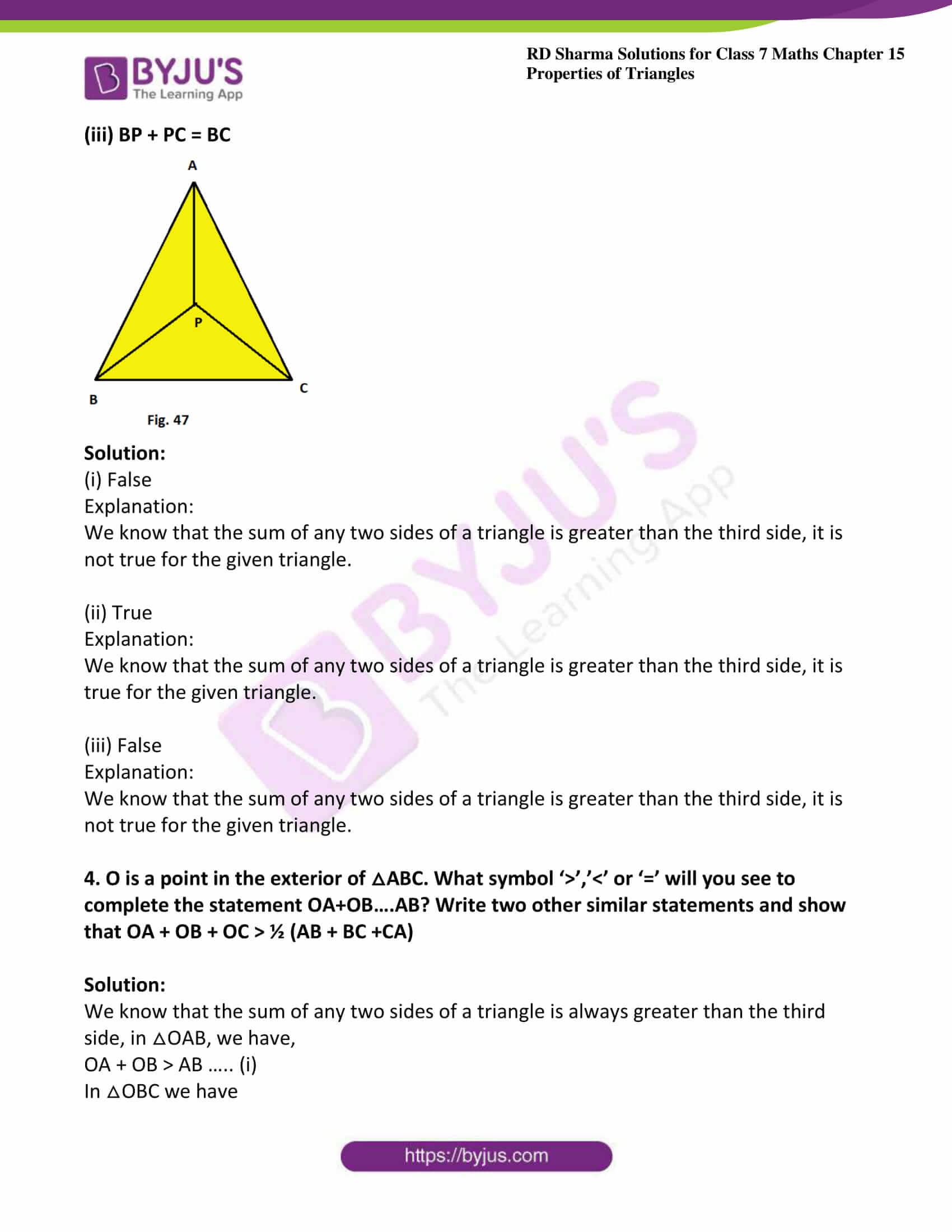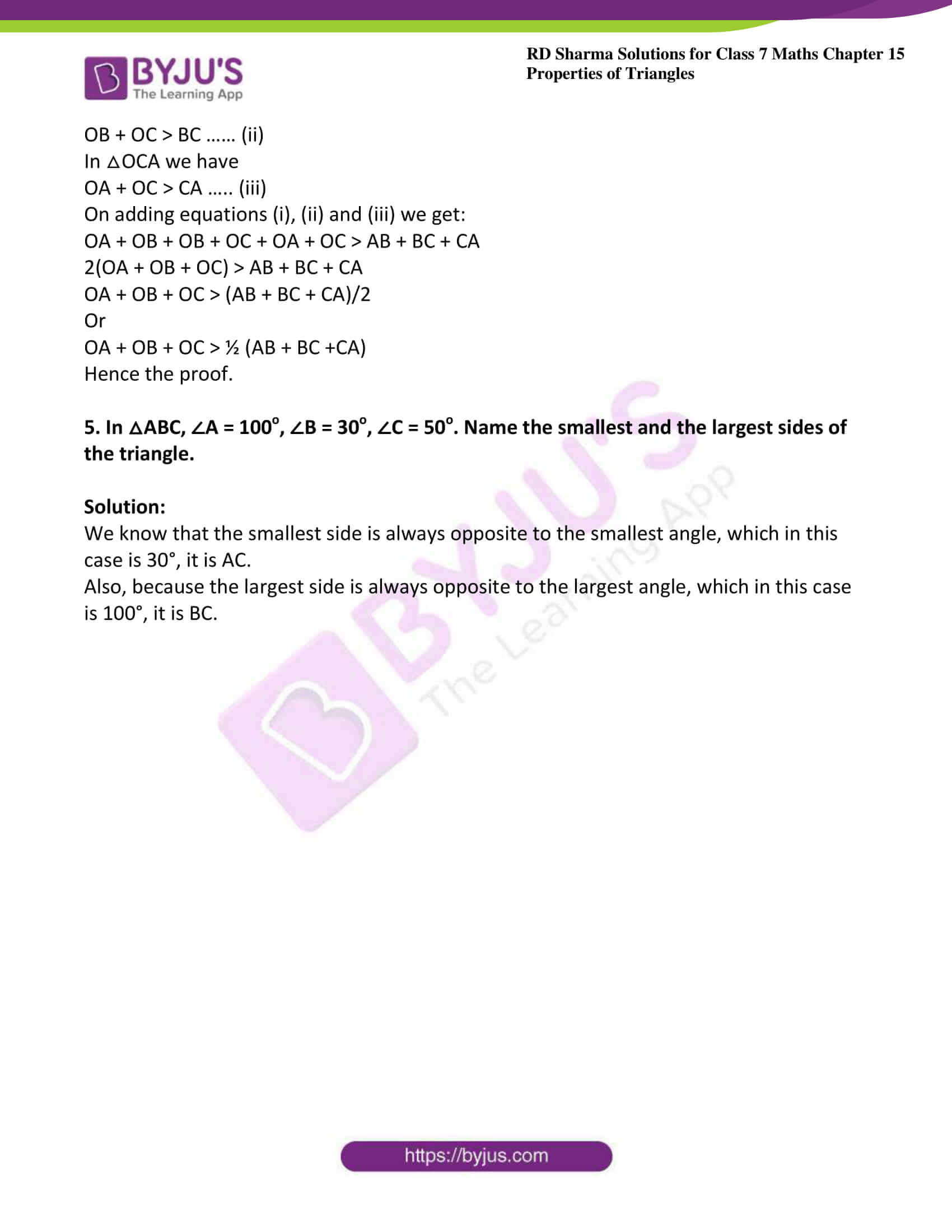### Access answers to Maths RD Sharma Solutions For Class 7 Chapter 15 – Properties of Triangles Exercise 15.4

Exercise 15.4 Page No: 15.24

1. In each of the following, there are three positive numbers. State if these numbers could possibly be the lengths of the sides of a triangle:

(i) 5, 7, 9

(ii) 2, 10, 15

(iii) 3, 4, 5

(iv) 2, 5, 7

(v) 5, 8, 20

Solution:

(i) Given 5, 7, 9

Yes, these numbers can be the lengths of the sides of a triangle because the sum of any two sides of a triangle is always greater than the third side.

Here, 5 + 7 > 9, 5 + 9 > 7, 9 + 7 > 5

(ii) Given 2, 10, 15

No, these numbers cannot be the lengths of the sides of a triangle because the sum of any two sides of a triangle is always greater than the third side, which is not true in this case.

Here, 2 + 10 < 15

(iii) Given 3, 4, 5

Yes, these numbers can be the lengths of the sides of a triangle because the sum of any two sides of triangle is always greater than the third side.

Here, 3 + 4 > 5, 3 + 5 > 4, 4 + 5 > 3

(iv) Given 2, 5, 7

No, these numbers cannot be the lengths of the sides of a triangle because the sum of any two sides of a triangle is always greater than the third side, which is not true in this case.

Here, 2 + 5 = 7

(v) Given 5, 8, 20

No, these numbers cannot be the lengths of the sides of a triangle because the sum of any two sides of a triangle is always greater than the third side, which is not true in this case.

Here, 5 + 8 < 20

2. In Fig. 46, P is the point on the side BC. Complete each of the following statements using symbol ‘=’,’ > ‘or ‘< ‘so as to make it true:

(i) AP… AB+ BP

(ii) AP… AC + PC

(iii) AP…. ½ (AB + AC + BC)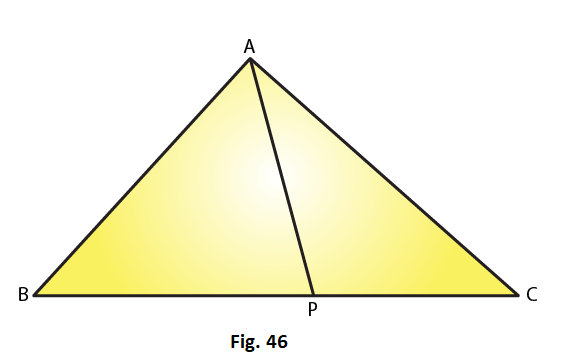Solution:

(i) In △APB, AP < AB + BP because the sum of any two sides of a triangle is greater than the third side.

(ii) In △APC, AP < AC + PC because the sum of any two sides of a triangle is greater than the third side.

(iii) AP < ½ (AB + AC + BC)

In △ABP and △ACP, we can write as

AP < AB + BP… (i) (Because the sum of any two sides of a triangle is greater than the third side)

AP < AC + PC … (ii) (Because the sum of any two sides of a triangle is greater than the third side)

On adding (i) and (ii), we have:

AP + AP < AB + BP + AC + PC

2AP < AB + AC + BC (BC = BP + PC)

AP < ½ (AB + AC + BC)

3. P is a point in the interior of △ABC as shown in Fig. 47. State which of the following statements are true (T) or false (F):

(i) AP + PB < AB

(ii) AP + PC > AC

(iii) BP + PC = BC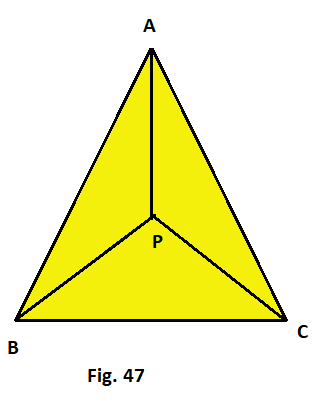Solution:

(i) False

Explanation:

We know that the sum of any two sides of a triangle is greater than the third side, it is not true for the given triangle.

(ii) True

Explanation:

We know that the sum of any two sides of a triangle is greater than the third side, it is true for the given triangle.

(iii) False

Explanation:

We know that the sum of any two sides of a triangle is greater than the third side, it is not true for the given triangle.

4. O is a point in the exterior of △ABC. What symbol ‘>’,’<’ or ‘=’ will you see to complete the statement OA+OB….AB? Write two other similar statements and show that OA + OB + OC > ½ (AB + BC +CA)

Solution:

We know that the sum of any two sides of a triangle is always greater than the third side, in △OAB, we have,

OA + OB > AB ….. (i)

In △OBC we have

OB + OC > BC …… (ii)

In △OCA we have

OA + OC > CA ….. (iii)

On adding equations (i), (ii) and (iii) we get:

OA + OB + OB + OC + OA + OC > AB + BC + CA

2(OA + OB + OC) > AB + BC + CA

OA + OB + OC > (AB + BC + CA)/2

Or

OA + OB + OC > ½ (AB + BC +CA)

Hence the proof.

5. In △ABC, ∠A = 100o, ∠B = 30o, ∠C = 50o. Name the smallest and the largest sides of the triangle.

Solution:

We know that the smallest side is always opposite to the smallest angle, which in this case is 30°, it is AC.

Also, because the largest side is always opposite to the largest angle, which in this case is 100°, it is BC.